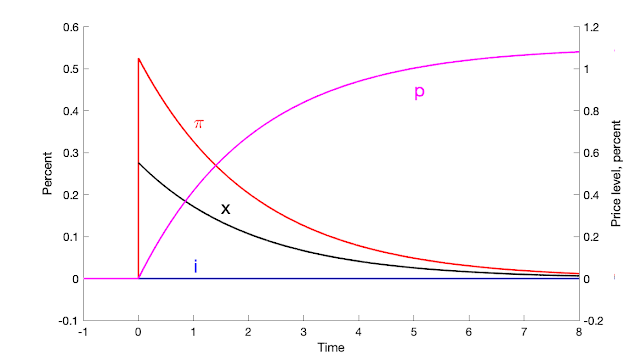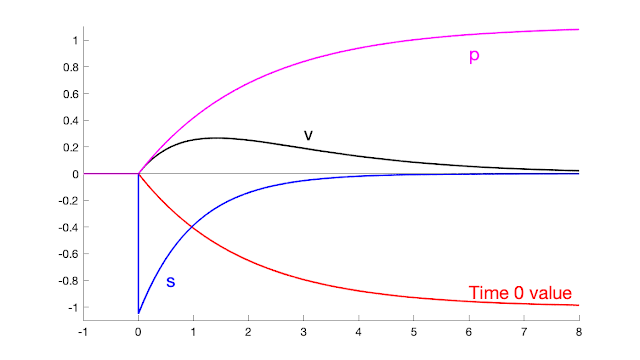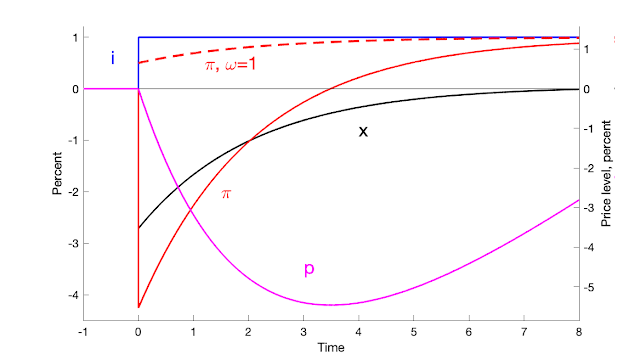## Thursday, August 25, 2022

### WSJ inflation stability oped -- with pictures

"Nobody Knows How Interest Rates Affect Inflation" is a new oped in the Wall Street Journal August 25. (Full version will be posted here Sept 25). It's a distillation of two recent essays, Expectations and the Neutrality of Interest Rates and Inflation Past, Present, and Future and some recent talks.

The Fed has only very slowly raised interest rates in response to inflation:

Does the Fed's slow reaction mean that inflation will spiral away, until the Fed raises interest rates above inflation? The traditional theory says yes. In this theory, inflation is unstable when the Fed follows an interest rate target. Unstable:
In this view, the Fed must promptly raise interest rates above inflation to contain inflation, as the seal must move its nose more than one for one to get back under the ball. Until we get interest rates of 9% and more inflation will spiral upward.

The view comes fundamentally from adaptive expectations. Inflation = expected inflation - (effect of high real interest rates, i.e. interest rate - expected inflation). If expected inflation is last year's inflation, you can see inflation spirals up until the real interest rate is positive.

But an alternative view says that inflation is fundamentally stable. Stable:

Econometric tests aren't that useful -- inflation and interest rates move together in the long run in both views, and jiggle around each other. Episodes are salient. The unstable view points to the 1970s:

The Fed reacted too slowly to shocks, the story goes, letting inflation spiral up until it finally got its nose under the ball in 1980, driving inflation back down again. But the stable view points to the 2010s:

It's exactly the opposite situation. Deflation broke out. The Fed could not move interest rates below zero. The classic analysis screamed "here comes the deflation spiral." It didn't happen. Wolf. If the deflation spiral did not break out, why will an inflation spiral break out now?

Not elsewhere: As I look at these, I start to have additional doubts about the classic story of the 1970s. The Fed did move interest rates one for one. Inflation did not simply spiral out of control. For example, note in 1975, inflation did come right back down again, even though interest rates never got above inflation. The simple spiral story does not explain why 1975 was briefly successful. Each of the waves of inflation also was sparked by new shocks.

Who is right? I don't pound my fist on the table, but stability, at least in the long run, is looking more and more likely to me. That says inflation will eventually go away, after the price level has risen enough to inflate away the recent debt blowout, even if the Fed never raises rates above inflation. In any case, it looks like we have another really significant episode all set up, to add to the 1970s and 2010s. If inflation spirals, stability is in trouble. If inflation fades away and interest rates never exceed inflation, the classic view is really in trouble. The ingredients are stirred, the test tube is on the table...

But, that's a conditional mean. A new shock could send inflation spiraling up again, just as may have happened in the 1970s. New virus, China invades Taiwan, financial crisis, sovereign debt crisis, another fiscal blowout -- if the US forgives college debt, why not forgive home loans? Credit cards? -- all could send inflation up again, and ruin this beautiful experiment.

## Tuesday, August 9, 2022

### Climate policy numbers

Most legislation or regulation that spends hundreds of billions of dollars aimed at a purpose is extensively analyzed or scored to that purpose. OK, the numbers are  often, er, a bit unreliable, but at least proponents go through the motions and lay out assumptions one can examine and calculate differently. Tax and spending laws come with extensive analysis of just how much the government will make or spend. This is especially true when environment is concerned. Building anything requires detailed environmental assessments. An environmental review typically takes 4.5 years before the lawsuits begin.

In this context, I'm amazed that climate policy typically comes with no numbers, or at least none that I can find readily available in major media. We're going to spend an additional \$250 billion or so on climate policies in the humorously titled "inflation reduction act." OK,  how much carbon will that remove, on net, all things included, how much will that lower the temperature and when, how much and when will it quiet the rise of the oceans?

Finally, I have seen one number, advertised in the Wall Street Journal

Our contributor Bjorn Lomborg looked at the Rhodium Group estimate for CO2 emissions reductions from Schumer-Manchin policies. He then plugged them into the United Nations climate model to measure the impact on global temperature by 2100. He finds the bill will reduce the estimated global temperature rise at the end of this century by all of 0.028 degrees Fahrenheit in the optimistic case. In the pessimistic case, the temperature difference will be 0.0009 degrees Fahrenheit.

## Saturday, August 6, 2022

### The Fiscal Theory of Inflation

We often summarize that the fiscal theory is a theory of the price level: The price level adjusts so that the real value of government debt equals the present value of surpluses. That characterization seems to leave it to a secondary role. But with any even tiny price stickiness, fiscal theory is really a fiscal theory of inflation. The following two parables should make the point, and are a good starting point for understanding what fiscal theory is really all about. This point is somewhat buried in Chapter 5.7 of Fiscal Theory of the Price Level

Start with the response of the economy to a one-time fiscal shock, a 1% unexpected decline in the sum of current and expected future surpluses, with no change in interest rate, at time 0. The model is below, but today's point is intuition, not staring at equations. This is the continuous-time version of the model, which clarifies the intuitive points.Response to 1% fiscal shock at time 0 with no change in interest rates

The one-time fiscal shock produces a protracted inflation. The price level does not move at all on the date of the shock. Bondholders lose value from an extended period of negative real interest rates -- nominal interest rates below inflation.

What's going on? The government debt valuation equation with instantaneous debt and with perfect foresight is $V_{t}=\frac{B_{t}}{P_{t}}=\int_{\tau=t}^{\infty}e^{-\int_{w=t}^{\tau}\left( i_{w}-\pi_{w}\right) dw}s_{\tau}d\tau$ where $$B$$ is the nominal amount of debt, $$P$$ is the price level, $$i$$ is the interest rate $$\pi$$ is inflation and $$s$$ are real primary surpluses. We discount at the real interest rate $$i-\pi$$. We can use this valuation equation to understand variables before and after a one-time probability zero "MIT shock."

With flexible prices, we have a constant real interest rate, so $$i_w-\pi_w$$. Thus if there is a downward jump in  $$\int_{\tau=t}^{\infty}e^{-r \tau}s_{\tau}d\tau$$, as I assumed to make the plot, then there must be an upward jump in the price level $$P_t$$, to devalue outstanding debt. (Similarly, a diffusion component to surpluses must be matched by a diffusion component in the price level.) The initial price level adjusts so that the real value of debt equals the present value of surpluses. This is the standard understanding of the fiscal theory of the price level. Short-term debt holders cannot be made to lose from expected future inflation.

But that's not how the simulation in the figure works, with sticky prices. Since now both $$B_t$$ and $$P_t$$ on the left hand side of the government debt valuation equation cannot jump, the left-hand side itself cannot jump. Instead, the government debt valuation equation determines which path of inflation $$\{\pi_w\}$$ which, with the fixed nominal interest rate $$i_w$$, generates just enough lower real interest rates $$\{i_w-\pi_w\}$$ so that the lower discount rate just offsets the lower surplus.  Short-term bondholders lose value as their debt is slowly inflated away during the period of low real interest rates, not in an instantanoues price level jump.

In this sticky-price model, the price level cannot jump or diffuse because only an infinitesimal fraction of firms can change their price at any instant in time. The price level is continuous and differentiable. The inflation rate can jump or diffuse, and it does so here; the price level starts rising. As we reduce price stickiness, the price level rise happens faster, and smoothly approaches the limit of a price-level jump for flexible prices.

In short, fiscal theory does not operate by changing the initial price level. Fiscal theory determines the path of the inflation rate. It really is a fiscal theory of inflation, of real interest rate determination.

The frictionless model remains a guide to how the sticky price model behaves in the long run. In the frictionless model, monetary policy sets expected inflation via $$i_t = r+E_t \pi_{t+1}$$ or $$i_t = r+\pi_t$$, while fiscal policy sets unexpected inflation $$\pi_{t+1}-E_t\pi_{t+1}$$ or $$dp_t/p_t-E_t dp_t/p_t$$.  In the long run of my simulation, the price level does inexorably rise to devalue debt, and the interest rate determines the long-run expected inflation. But this long-run characterization does not provide useful intuition for the higher frequency path, which is what we typically want to  interpret and analyze.

It is a better characterization of these dynamics that monetary policy---the nominal interest rate---determines a set of equilibrium inflation paths, and fiscal policy determines which one of these paths is the overall equilibrium, inflating away just enough initial debt to match the decline in surpluses.Response to 1% deficit shock at time 0 with no change in interest rate

This second graph gives a bit more detail of the fiscal-shock simulation, plotting the primary surplus $$s$$, the value of debt $$v$$, and the price level $$p$$. The surplus follows an AR(1). The persistence of that AR(1) is irrelevant to the inflation path. All that matters is the initial shock to the discounted stream of surpluses. (I make a big fuss in FTPL that you should not use AR(1) surplus process to match fiscal data, since most fiscal shocks have an s-shaped response, in which deficits correspond to larger surpluses. However, it is still useful to use an AR(1) to study how the economy responds to that component of the fiscal shock that is not repaid.)

To see how initial bondholders end up financing the deficits, track the value of those bondholders' investment, not the overall value of debt. The latter includes debt sales that finance deficits. The real value of a bond investment held at time 0, $$\hat{v}$$, follows $d \hat{v}_t = (r \hat{v}_t + i_t - \pi_t)dt.$ I plot the time-zero value of this portfolio, $e^{-rt} \hat{v}_t.$ As you can see this value smoothly declines to -1%. This is the quantity that matches the 1% by which surpluses decline. (I picked the initial surplus shock $$d\varepsilon_{s,t}=1/(r+\eta_s)$$ so that $$\int_{\tau=0}^\infty e^{-r\tau}\tilde{s}_t d\tau =-1.$$ )Response to interest rate shock at time 0 with no change in surpluses

The third graph presents the response to an unexpected permanent rise in interest rate. With long-term debt, inflation initially declines. The Fed can use this temporary decline to offset some fiscal inflation. Inflation eventually rises to meet the interest rates. Most interest rate rises are not permanent, so we do not often see this long-run stability or neutrality property. The initial decline in interest rates comes in this model from long-term debt. As the dashed line shows, with shorter-maturity debt inflation  rises right away. With instantaneous debt, inflation follows the interest rate exactly.

Again, in this continuous-time model the price level does not move instantly. The higher interest rate sets off a period of lower inflation, not a price-level drop.

With long-term debt the perfect-foresight valuation equation is $V_{t}=\frac{Q_tB_{t}}{P_{t}}=\int_{\tau=t}^{\infty}e^{-\int_{w=t}^{\tau}\left( i_{w}-\pi_{w}\right) dw}s_{\tau}d\tau.$ where $$Q_t$$ is the nominal price of long-term government debt. Now, with flexible prices, the real rate is fixed $$i_w=\pi_w$$. With no change in surplus $$\{s_\tau\}$$, the right hand side cannot change. Inflation $$\{\pi_w\}$$ then simply follows the AR(1) pattern of the interest rate. However, the higher nominal interest rates induce a downward jump or diffusion in the bond price $$Q_t$$. With $$B_t$$ predetermined, there must be a downward jump or diffusion in the price level $$P_t$$. In this way, even with flexible prices, with long-term debt we can see an instant in which higher interest rates lower inflation before long run'' neutrality kicks in.

How does the price level not jump or diffuse with sticky prices? Now $$B_t$$ and $$P_t$$ are predetermined on the left hand side of the valuation equation. Higher nominal interest rates $$\{i_w\}$$ still drive a downward jump or diffusion in the bond price $$Q_t$$. With no change in $$s_\tau$$, a spread $$i_w-\pi_w$$ must open up to match the downward jump in bond price $$Q_t$$, which is what we see in the simulation. Rather than an instant downward jump in price level, there is instead a long period of low inflation, of slow price level decline, followed by a gradual increase in inflation.

Again, the frictionless model does provide intuition for the long-run behavior of the simulation. The three year decline in price level is reminiscent of the downward jump; the eventual rise of inflation to match the interest rate is reminiscent of the immediate rise in inflation. But again, in the actual dynamics we really have a theory of \emph{inflation}, not a theory of the \emph{price level}, as on impact the price level does not jump at all. Again, the valuation equation generates a path of inflation, of the real interest rate, not a change in the value of the initial price level.

The general lessons of these two simple exercises remain:

Both monetary and fiscal policy drive inflation. Inflation is not always and everywhere a monetary phenomenon, but neither is it always and everywhere fiscal.

In the long run, monetary policy completely determines the expected price level. As the inflation rate ends up matching the interest rate, inflation will go wherever the Fed sends it. If the interest rate went below zero (these are deviations from steady state, so that is possible), it would drag inflation down with it, and the price level would decline in the long run.

One can view the current situation as the lasting effect of a fiscal shock, as in the first graph. One can view the Fed's option to restrain inflation as the ability to add the dynamics of the second graph.

Don't be too put off by the simple AR(1) dynamics. First, these are responses to a single, one-time shock. Historical episodes usually have multiple shocks. Especially when we pick an episode ex-post based on high inflation, it is likely that inflation came from several shocks in a row, not a one-time shock. Second, it is relatively easy to add hump-shaped dynamics to these sorts of responses, by standard devices such as habit persistence preferences or capital accumulation with adjustment costs. Also, full models have additional structural shocks, to the IS or Phillips curves here for example. We analyze history with responses to those shocks as well, with policy rules that react to inflation, output, debt, etc.

The model  I use for these simple simulations is a simplified version of the model presented in FTPL 5.7.

\begin{aligned}E_t dx_{t} & =\sigma(i_{t}-\pi_{t})dt \\E_t d\pi_{t} & =\left( \rho\pi_{t}-\kappa x_{t}\right) dt\ \\ dp_{t} & =\pi_{t}dt \\ E_t dq_{t} & =\left[ \left( r+\omega\right) q_{t}+i_{t}\right] dt \\ dv_{t} & =\left( rv_{t}+i_{t}-\pi_{t}-\tilde{s}_{t}\right) dt+(dq_t - E_t dq_t) \\ d \tilde{s}_{t} & = -\eta_{s}\tilde{s}_{t}+d\varepsilon_{s,t} \\ di_{t} & = -\eta_{i}i_t+d\varepsilon_{i,t}. \end{aligned}

I use parameters $$\kappa = 1$$, $$\sigma = 0.25$$, $$r = 0.05$$, $$\rho = 0.05$$, $$\omega=0.05$$, picked to make the graphs look pretty. $$x$$ is output gap, $$i$$ is nominal interest rate, $$\pi$$ is inflation, $$p$$ is price level, $$q$$ is the price of the government bond portfolio, $$\omega$$ captures a geometric structure of government debt, with face value at maturity $$j$$ declining at $$e^{-\omega j}$$, $$v$$ is the real value of government debt, $$\tilde{s}$$ is the real primary surplus scaled by the steady state value of debt, and the remaining symbols are parameters.

Thanks much to Tim Taylor and Eric Leeper for conversations that prompted this distillation, along with evolving talks.

## Friday, August 5, 2022

### China game theory

In the last Goodfellows, we batted around the question of how China would respond to Nancy Pelosi's visit.

In this context, news just came in, announcing responses that we had not thought of: China is giving the US a big middle finger on climate. The announcement, from the Ministry of Foreign affairs, is short and clear:

In disregard of China’s strong opposition and serious representations, Speaker of the U.S. House of Representatives Nancy Pelosi visited China’s Taiwan region. On 5 August, the Ministry of Foreign Affairs announced the following countermeasures in response:

1.Canceling China-U.S. Theater Commanders Talk.

2.Canceling China-U.S. Defense Policy Coordination Talks (DPCT).

3.Canceling China-U.S. Military Maritime Consultative Agreement (MMCA) meetings.

4.Suspending China-U.S. cooperation on the repatriation of illegal immigrants.

5.Suspending China-U.S. cooperation on legal assistance in criminal matters.

6.Suspending China-U.S. cooperation against transnational crimes.

7.Suspending China-U.S. counternarcotics cooperation.

8.Suspending China-U.S. talks on climate change.

My emphasis. Slip the knife in at the end. (The others are also interesting.)